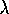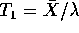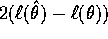#### STAT 450

Problems: Assignment 7

1. Page 398. Q 1.

2. Page 398 Q 2.

3. Page 398. Q 6.

4. Suppose X is an exponential().

1. Find the shortest exact 95% confidence interval based on.

2. Find the exact confidence level of 95% confidence intervals based on the chi-squared approximation to the distribution of the likelihood ratio. Compare the results with that of part a) based on length and coverage probabilities. Which method is better?

Richard Lockhart
Tue Nov 19 00:08:09 PST 1996